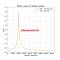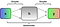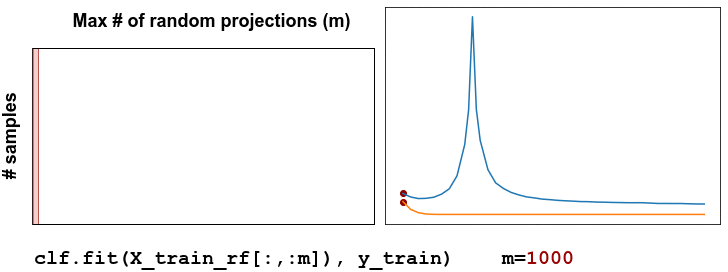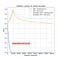# Recover the Double Descent Curve with an OPUPont des Arts, Paris. Picture courtesy of K. Yuan.

In an earlier blog post, we investigated the Double Descent phenomenon [1, 2]: a reconciliation of the classical underparametrized regime with the modern overparametrized one. Indeed, classical prescriptions in statistics lead to a sweet point, where the bias-variance tradeoff is balanced. In contrast, modern architectures contain millions of parameters that are optimized to achieve the best possible accuracy on training data. With so many parameters, one could expect overfitting. Yet, these architectures better generalize than classical methods.

Their unforeseen success still lacks a solid mathematical explanation. The double descent curve is a promising attempt to understand this phenomenon. Since the publication of the original paper , this topic has raised much interest in academia: more than ten papers have been published on the subject in the last three months only. A common feature of many of these papers [2, 3, 4] is the use of random projections. Indeed, they are key in easily changing the model complexity.

LightOn has developed an Optical Processing Unit (OPU) well suited to random projections computations . In some tasks our co-processor considerably reduces training time without deteriorating the accuracy of the model when compared with a GPU.Figure 1: double descent curve on MNIST using 10.000 fixed training samples and varying the number of random features. The interpolation point lies where the number of data points equals the number of random features, i.e. the model complexity.

How can we observe this phenomenon? A two-layer neural network is enough, where the first layer is fixed and drawn from a complex-valued random Gaussian distribution. Using the OPU to calculate the random projections, we can easily reproduce the double descent behavior.

In this tutorial we are going to see how to recover the double descent curve using LightOn’s OPU. In particular we will study, step by step, the code that generated the plot in Figure 1.

# Recovering the double descent curve:

1. Prepare your dataset: binarize the data before feeding them to the OPU;
2. Randomly project the data: tune the model complexity by tuning the number of random projections;
3. Classification: compute the train and test accuracy to recover the double descent curve.Figure 2: a simple representation of an autoencoder (AE). Its goal is to recover the input x after the hidden layer h. In our case h is a binary representation of the input x. Thus the AE learns how to encode the input data into a binary representation and then how to recover the original input from it. At the end of the training, we are interested only in the encoder function of the AE.

The input of the OPU must be binary. To transform the data into a binary representation, we can use a variety of binary encoding schemes. In this tutorial, we are going to use an autoencoder (AE). AEs are neural networks trained to reconstruct their input data to their output while learning a useful hidden representation of the data in the process. In our case, we desire our AE to learn a binary representation of the data. We can use an architecture like the following one:

This is a simple AE with one convolutional layer as encoder and one as decoder; a sketch of this architecture is shown in Figure 2. Its hyperparameters need to be tuned for the specific dataset, however the default values for the convolutions used in the code above offer satisfying performance on MNIST and CIFAR10. To enforce a binary representation we use a tanh function controlled by a parameter beta. During the training, we can increase beta until tanh(beta * x) converges to a sign function. Now we can train the AE with a simple reconstruction loss like the mean-squared error:

`loss = nn.MSELoss(y, x)`

where `y` is the reconstructed input. Once the training is over we can binarize the data by isolating the encoder and replacing the tanh function with a sign function:

`X_train_binary = ae.encode(X_train).view(X_train.shape,-1)X_test_binary = ae.encode(X_test).view(X_test.shape,-1)`

Here we binarized the data and reshaped them into one dimensional vectors. The reshaping happens without loss of information, since the 2-dimensional structure of the data is not critical when performing a random projection. Indeed, it would be like applying a permutation to a random matrix: it’s still a random matrix!

Randomly project the data

To obtain a double descent curve we need to explore the area before and beyond the so-called interpolation point, where the number of parameters equals the number of data points. It is important to increase the density of points in the proximity of the peak. There is no need to iteratively project the data for each given number of random features; we can project them once on the maximum number of random features we are going to use. Smaller random projections (RPs) can be recovered by slicing the largest one. The procedure is explained by the animation in Figure 3.

Here we define the list of random features values we will use in the classification part. Afterwards we project the train and test data. Using the OPU takes only a couple of lines of code. We instantiate the OPU class with `opu = OPU(n_components=max_rps)`. Then, we perform the random projection of the input matrix with `opu.transform(X_train_binary).`The OPU accepts and returns both `numpy.ndarray` and `torch.Tensor`. To prevent the OPU from being opened and closed twice we used the context manager `with opu:`. The context manager might not be necessary with new version of lightonopu.Figure 3: when we project our data on the maximum number of random projections we obtain a number of samples x max_rps matrix. We can explore the various points of the double descent curve by using slices of this matrix.

Classification

Let’s choose a linear classifier, such as the RidgeClassifier. We can now train it on the various slices of the projected data.

Where the various slices are taken from zero to the desired number of random features. Now the only thing left is is to plot the results to display the curve shown in Fig. 1.

Tips & Tricks

1. Using a different classifier: the interpolation point can vary depending on the classifier used. A ridge classifier uses a one-vs-all approach and the interpolation point will lie where the number of parameters equals the number of data points. A multinomial logistic regression instead will have an interpolation point at the number of data points x number of classes. For example, the interpolation point for MNIST (60k data points) lies at 60k random features using a ridge classifier and at 600k random features using a logistic classifier.
2. To average the results on multiple trials there is a smarter choice than repeating the above process for multiple trials. We directly project on: max_rps * N_trials.

At each iteration we randomly select the desired number of random features. By doing that we can randomly project the data once instead of `N_trials` times. This is possible only on hardware that can reach really large output dimensions like the OPU. In fact using Fig 1 as reference: `N_trials=10` would mean for example to project the data on 400.000 RPs.

# ConclusionFigure 4: double descent curve on CIFAR10 using 10.000 fixed training samples and varying the number of random features.

In this blog post, we learned how to recover the double descent curve using the LightOn’s OPU. This curve can be tricky to observe with a large number of data points without dedicated hardware.

In Figure 4 we have adapted the code just discussed to recover the double descent curve on CIFAR10. It is clear how the OPU does not deteriorate the accuracy with respect to the GPU (quite the opposite in this figure!)

Don’t wait to save your work anymore, come and try our OPU through LightOn Cloud, a simple platform, made for you to develop your Machine Learning models. Apply now to use the LightOn Cloud.

If you want to check out the code and run it, here is the link to the Jupyter notebook.

# About us

LightOn is a hardware company that develops new optical processors that considerably speed up big data computation. LightOn’s processors open new horizons in computing and engineering fields that are facing computational limits. Interested in speeding your computations up? Try out our solution on LightOn Cloud ! 🌈

Follow us on Twitter at @LightOnIO , subscribe to our newsletter and register to our workshop series. We live stream, so you can join from anywhere. 🌍

# The author

Alessandro Cappelli, Machine Learning Engineer at LightOn AI Research.

# Acknowledgement

Thanks to Igor Carron, Julien Launay, Victoire Louis and Iacopo Poli for reviewing this blog post.

# References

 Geiger, Mario, et al. “Jamming transition as a paradigm to understand the loss landscape of deep neural networks.” Physical Review E 100.1 (2019): 012115.

 Belkin, Mikhail, et al. “Reconciling modern machine-learning practice and the classical bias–variance trade-off.” Proceedings of the National Academy of Sciences 116.32 (2019): 15849–15854.

 Mei Song, and Andrea Montanari. “The generalization error of random features regression: Precise asymptotics and double descent curve.” arXiv preprint arXiv:1908.05355 (2019).

 D’Ascoli, Refinetti, et al. “Double Trouble in Double Descent : Bias and Variance(s) in the Lazy Regime.”

 Saade, Alaa, et al. “Random projections through multiple optical scattering: Approximating kernels at the speed of light.” 2016 IEEE International Conference on Acoustics, Speech and Signal Processing (ICASSP). IEEE, 2016.

We are a technology company developing Optical Computing for Machine Learning. Our tech harvests Computation from Nature, We are at lighton.ai

We are a technology company developing Optical Computing for Machine Learning. Our tech harvests Computation from Nature, We are at lighton.ai